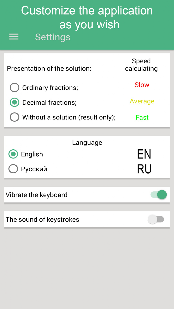Matrix Operations

Solution of systems of linear equations by the Jordan-Gauss method

-Solution of systems of linear equations by the Cramer method
-Solution of systems of linear equations by the Gauss method
-Finding the determinant of the matrix
-Calculation of the transpose matrix
-Matrix multiplication by number
-Erection of a matrix in degree
-Finding the inverse matrix
-Matrix rank calculation
-Matrix multiplication
-Matrix subtraction
All solutions are detailed, step by step

What’s New
Added the representation of solutions in the form of ordinary fractions;
Added settings window. You can configure the following parameters in it:
– Presentation of the result in the form of:
1) Ordinary fractions;
2) Decimal fractions;
3) Without solution (only result);
– Keyboard settings:
1) Enable/disable vibration feedback;
2) Enable/disable the click sound;
– Change interface language

Screenshots

•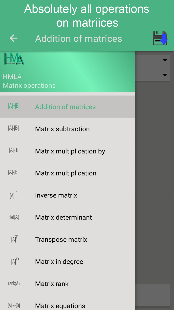•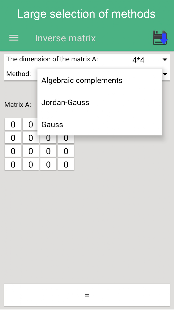••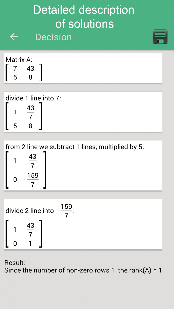•••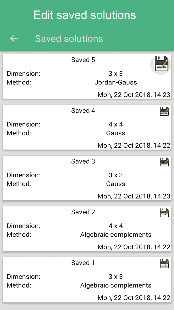•